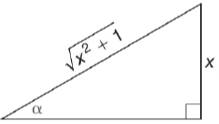Chapter 11.3, Problem 9EElementary Geometry For College St...

7th Edition
Alexander + 2 others
ISBN: 9781337614085

Solutions

Chapter
SectionElementary Geometry For College St...

7th Edition
Alexander + 2 others
ISBN: 9781337614085
Textbook Problem

In Exercises 5 to 10, find the value (or expression) for each of the six trigonometric ratios of angle α . Use the Pythagorean Theorem as needed.To determine

To find:

The value for each of the six trigonometric ratios of angle α.

Explanation

Given:

The given figure is

Definition used:

The six trigonometric ratios:

Calculation:

Let the unknown side in the triangle be a.

In a right triangle, (hypotenuse)2=(opposite)2+(adjacent)2

(x2+1)2=x2+(a)2x2+1=x2+a21=a21=a21=a

Thus we have

From the figure, opposite=x, adjacent=1, hypotenuse=x2+1

Still sussing out bartleby?

Check out a sample textbook solution.

See a sample solution

The Solution to Your Study Problems

Bartleby provides explanations to thousands of textbook problems written by our experts, many with advanced degrees!

Get Started

Find more solutions based on key concepts